Resource Title search

Text Search of NRICH resource titles

Or find resource begining with:Age 14 to 16 Challenge Level: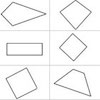Age 7 to 11 Challenge Level:

A task which depends on members of the group noticing the needs of others and responding.Age 16 to 18 Short Challenge Level:

Can you solve this problem involving powers and quadratics?Age 14 to 16 Challenge Level:

Given a square ABCD of sides 10 cm, and using the corners as centres, construct four quadrants with radius 10 cm each inside the square. The four arcs intersect at P, Q, R and S. Find the. . . .Age 16 to 18 Challenge Level:

Find all positive integers a and b for which the two equations: x^2-ax+b = 0 and x^2-bx+a = 0 both have positive integer solutions.Age 11 to 14 Challenge Level:

Surprising numerical patterns can be explained using algebra and diagrams...Age 14 to 16 Short Challenge Level:

The curve $y=x^2−6x+11$ is rotated through $180^\circ$ about the origin. What is the equation of the new curve?Age 16 to 18 Challenge Level:

A lune is the area left when part of a circle is cut off by another circle. Can you work out the area?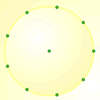Age 7 to 11 Challenge Level:

How many DIFFERENT quadrilaterals can be made by joining the dots on the 8-point circle?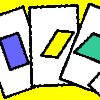Age 11 to 14 Challenge Level:

A game for 2 or more people, based on the traditional card game Rummy. Players aim to make two `tricks', where each trick has to consist of a picture of a shape, a name that describes that shape, and. . . .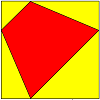Age 11 to 14 Challenge Level:Age 11 to 16 Challenge Level:

Four numbers on an intersection that need to be placed in the surrounding cells. That is all you need to know to solve this sudoku.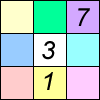Age 11 to 16 Challenge Level:

Four small numbers give the clue to the contents of the four surrounding cells.Quantum Scholars at the University of Roehampton

Resources to accompany Charlie's workshop with teachers from overseas.Quarters

Age 14 to 16 Short Challenge Level:

Weekly Problem 27 - 2014
Four congruent isosceles trapezia are placed in a square. What fraction of the square is shaded?Quarters, Quarters

Age 7 to 11 Challenge Level:

An activity causing pupils to seriously consider and create ways of quartering shapes.Quartics

Age 16 to 18 Challenge Level:

Investigate the graphs of y = [1 + (x - t)^2][1 + (x + t^)2] as the parameter t varies.Quaternions and Reflections

Age 16 to 18 Challenge Level:

See how 4 dimensional quaternions involve vectors in 3-space and how the quaternion function F(v) = nvn gives a simple algebraic method of working with reflections in planes in 3-space.Quaternions and Rotations

Age 16 to 18 Challenge Level:

Find out how the quaternion function G(v) = qvq^-1 gives a simple algebraic method for working with rotations in 3-space.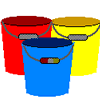Question De Chaudières

Age 7 to 11 Challenge Level:

"Buckets Of Thinking" en FrançaisQuestion of Three Sides

Age 14 to 16 Short Challenge Level:

Can you find the length of the third side of this triangle?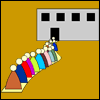Queue

Age 5 to 7 Challenge Level:

25 students are queuing in a straight line. How many are there between Julia and Jenny?Queueing

Age 3 to 5 Challenge Level:

This resource explores the rich mathematics in activities for young children involing queues.Quick Route

Age 16 to 18 Challenge Level:

What is the quickest route across a ploughed field when your speed around the edge is greater?Quick Sum

Age 16 to 18 Short Challenge Level:

Is this surd sum exactly 3?Quick Times

Age 11 to 14 Challenge Level:

32 x 38 = 30 x 40 + 2 x 8; 34 x 36 = 30 x 40 + 4 x 6; 56 x 54 = 50 x 60 + 6 x 4; 73 x 77 = 70 x 80 + 3 x 7 Verify and generalise if possible.Quick Ways In....

Age 5 to 11

teacher short articlesQuiz Question

Age 11 to 14 Short Challenge Level:

Can you work out how many quizzes have to be played before we have a winner?Quiz Questions

Age 11 to 14 Short Challenge Level:

Jack does a 20-question quiz. How many questions didn't he attempt?Quorum-sensing

Age 16 to 18 Short Challenge Level:

This problem explores the biology behind Rudolph's glowing red nose.